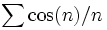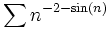# Math Techniques

### Number Theory

• Greatest common divisor, Least common multiple, Modular inverses, Extended Euclidean Algorithm, The Euclid Code
• Example Hyperbolic Quadratic Form (http://efnet-math.org/math_tech/HyperQuadForm.html) Solution
• Diophantine Equation (http://efnet-math.org/math_tech/dioph2.htm): 2a + 3b = 5c Not elementary

### Easy Analysis (not always serious)

• Tough Trig Series (http://www.efnet-math.org/math_tech/ToughTrig.html):$\sum \cos(n)/n$ and Really Tough Trig Series (http://www.efnet-math.org/math_tech/series5.pdf)$\sum n^{-2-\sin(n)}$
• Euler's Identity Expansion (http://www.efnet-math.org/Meta/e_x_times_cos_x.html) of excos(x)
• Finding the Sine of 1 Degree (http://www.efnet-math.org/Meta/sine1.htm)
• L'Hôpital Rulez